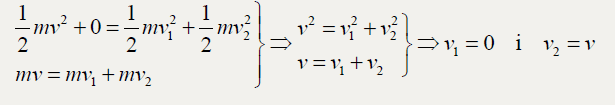# How is V1 deduced to be zero in the last step here?

• califauna
Yes, you could look at it that way, but I was thnking more in terms of everyday experience that solid objects cannot pass through each other.

## Homework Statement

A billiard ball moving at 2 m/s collides (0 degrees) with another of the same weight and at rest, in a perfectly elastic collision. Demonstrate with equations why the balls trade velocities.

## The Attempt at a Solution

In the provided explanation, I can understand what the parallel equations they set up represent, and how they reduce them by cancelling, but I can't see how the final logical step is made, where they deduce that V1 = 0,What does the lower (momentum) equation tell you about v2?

Plug v=v1+v2 into v2=v12+v22 and you will get 2v1v2 = 0. So either v1 =0 or v2 = 0

haruspex said:
What does the lower (momentum) equation tell you about v2?

That v^2 = (v1+v2)^2

FactChecker said:
Plug v=v1+v2 into v2=v12+v22 and you will get 2v1v2 = 0. So either v1 =0 or v2 = 0

When I do (v1+v2)2=v12+v22, after taking the root on both sides I get get v1+v2=v1+v2. What am I doing wrong?

califauna said:
When I do (v1+v2)2=v12+v22, after taking the root on both sides I get get v1+v2=v1+v2. What am I doing wrong?
You cannot take the square root of the right hand side like that. The square root of a sum is not the same as the sum of the square roots.
Instead, expand the left hand side.

haruspex said:
You cannot take the square root of the right hand side like that. The square root of a sum is not the same as the sum of the square roots.
Instead, expand the left hand side.
Ah yes. Ok, I am left with 2V1v2 = 0.

Obviously the struck ball is going to be moving so it cannot be the one which ends up with a velocity of zero, but is there any other logical or mathematical method of deducting which of the two balls will be the one which will have zero velocity? Something which the exam question might require demonstation of in order to get the full marks for the question?

califauna said:
Ah yes. Ok, I am left with 2V1v2 = 0.

Obviously the struck ball is going to be moving so it cannot be the one which ends up with a velocity of zero, but is there any other logical or mathematical method of deducting which of the two balls will be the one which will have zero velocity? Something which the exam question might require demonstation of in order to get the full marks for the question?
Both momentum and energy would be conserved if the first ball manages to pass straight through the stationary ball, but some other law may violated, do you think?

•FactChecker and califauna
haruspex said:
Both momentum and energy would be conserved if the first ball manages to pass straight through the stationary ball, but some other law may violated, do you think?

Not sure if the glitch in the matrix type clue about the balls passing through each other is a hint at this particlar law, but, Newtons third law regarding equal and opposite force?

Last edited:
califauna said:
Not sure if the glitch in the matrix type clue about the balls passing through each other is a hint at this particlar law, but, Newtons third law regarding equal and opposite force?
Yes, you could look at it that way, but I was thnking more in terms of everyday experience that solid objects cannot pass through each other. Quantum effects aside.

•califauna